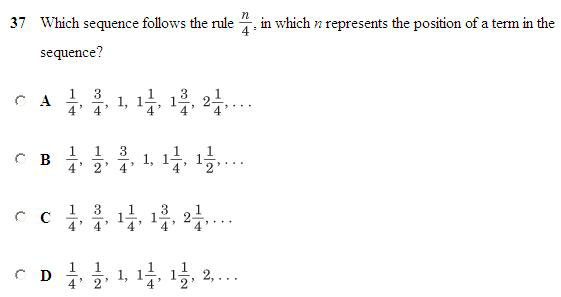SEARCH HOMEMath Central Quandaries & QueriesWhich sequence satisfies the rule n/4?Hi Shelly,

This is what you sentn represents the position of the term in the sequence. So the first term in the sequence is n=1, the second term is n=2, etc.

So just write out n/4 several times in a row. The first time, replace n with a "1", the second time replace it with a "2" and so on.

Reduce the fractions as you go into simplest terms. What answer matches?

Hope this helps,
Stephen La Rocque.Math Central is supported by the University of Regina and The Pacific Institute for the Mathematical Sciences.